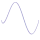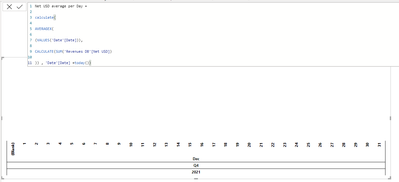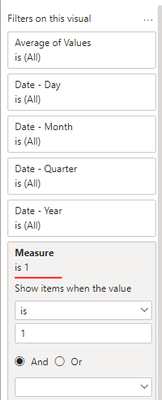cancel
Showing results for
Did you mean:Super User

## Bring only to the present day

Hello everyone!

And thanks to all the helpers

I have this formula:

Net USD average per Day =

AVERAGEX(

(VALUES('Date'[Date])),

CALCULATE(SUM('Revenues DB'[Net USD])

))

I want her to bring me data but only to the present day
And not the rest of the month as in the picture

What to do?

Attached is a link to my files:

https://1drv.ms/f/s!AonyYI-TdspHgUgReR5uqvnKLTCFThanks!

Mark my post as a solution!

1 ACCEPTED SOLUTIONCommunity Support

Hi @netanel ,

Net USD average per Day real one =
AVERAGEX(
(VALUES('Date'[Date])),
CALCULATE(SUM('Revenues DB'[Net USD])
))

you can just add calculate outside the formlua.

Net USD average per Day real one =
CALCULATE(
AVERAGEX(
(VALUES('Date'[Date])),
CALCULATE(SUM('Revenues DB'[Net USD])
)
),'Revenues DB'[Date]<TODAY())

Best Regards

Community Support Team _ chenwu zhu

12 REPLIES 12Super User

@netanel , Try like

Net USD average per Day =

calculate(

AVERAGEX(

(VALUES('Date'[Date])),

CALCULATE(SUM('Revenues DB'[Net USD])

)) , 'Date'[Date] =today())Super User

Hey @amitchandak

If you put your formula in the files I attached

you find that she's not working ...Mark my post as a solution!Super User

@netanel , try like

Avg of all dates on a filtered date, if you need less that that we need add logic

``````Net USD average per Day =
CALCULATE( AVERAGEX(
(VALUES('Date'[Date])),
CALCULATE(SUM('Revenues DB'[Net USD]),all('Date')
)),filter(('Date'),'Date'[Date]= TODAY()))``````Super User

I tried, now he brings me only the present day, and not the rest of the days backone more thing

When I change the sign to smaller from the current day all the information is the same,
Do you know why?Mark my post as a solution!Community Support

Hi @netanel ,

I have a menthod you can try. let's call it mark tag.

create a measure.

``````Measure =
IF( SELECTEDVALUE( calender[Date] ) > TODAY(), BLANK(), 1 )
``````

Then put the measure in the filters pane and set items as 1 as following screenshot show.Result:Pbix in the end you can refer.

Best Regards

Community Support Team _ chenwu zhu

If this post helps, then please consider Accept it as the solution to help the other members find it more quickly.Super User

The formula works great and indeed brings only to the present day in the graph.
But my formula in the Value column still calculates the minuses?
Because I can not put your formula in the Card so the calculation of the average comes out incorrect ...

Mark my post as a solution!Community Support

Hi @netanel ,

Can you share your pbix file without sensitive data if you need more help.

Best Regards

Community Support Team _ chenwu zhuSuper User

I also shared above at the beginning of the post, sharing again here:

https://1drv.ms/f/s!AonyYI-TdspHgUhwngZ6OKvpnSsc

The formula and idea work great on the graph, I just want them to work on the card as well
i dont now how to connect the two formulas

My Formula:

Net USD average per Day =
AVERAGEX(
(VALUES('Date'[Date])),
CALCULATE(SUM('Revenues DB'[Net USD])
))

Net Trying = if(SELECTEDVALUE('Date'[Date])> TODAY(),BLANK(),1)

Mark my post as a solution!Community Support

Hi @netanel ,

code:

``````Net USD average per Day created by chenwuz =
CALCULATE(
DIVIDE( SUM( 'Revenues DB'[Net USD] ), COUNTROWS( 'Revenues DB' ) ),
'Revenues DB'[Date] < TODAY()
)
``````

Result:Best Regards

Community Support Team _ chenwu zhu

If this post helps, then please consider Accept it as the solution to help the other members find it more quickly.Super User

Your formula is good, I corrected a bit that it would suit me better, you just miss something small in it
The formula divides by the number of days in the month of say January so it divides by 31
I want her to divide the number of days in a month only until the current day
For example we are on December 27th so you will only split in 27 days and not in 31st

Can it be done?

Net USD try =

CALCULATE(

DIVIDE( SUM( 'Revenues DB'[Net USD] ), COUNTROWS( 'Date' ) ),

'Revenues DB'[Date] < TODAY()

)

My previous formula divides by the number of days that have values and because on the 31st of the month I have a minus so if we are on the 27th of the month it will divide by 28 days
Because in 27 days there are values and also in 31

I literally go in circles with this Measure

previous formula:

Net USD average per Day =

AVERAGEX(

(VALUES('Date'[Date])),

CALCULATE(SUM('Revenues DB'[Net USD])

))

Thanks!!!

Mark my post as a solution!Community Support

Hi @netanel ,

Net USD average per Day real one =
AVERAGEX(
(VALUES('Date'[Date])),
CALCULATE(SUM('Revenues DB'[Net USD])
))

you can just add calculate outside the formlua.

Net USD average per Day real one =
CALCULATE(
AVERAGEX(
(VALUES('Date'[Date])),
CALCULATE(SUM('Revenues DB'[Net USD])
)
),'Revenues DB'[Date]<TODAY())

Best Regards

Community Support Team _ chenwu zhuSuper User

Thanks to everyone for the direction and guidance
It helped me a lot!

This is the right measure:

Net USD try  =
CALCULATE(
DIVIDE( SUM( 'Revenues DB'[Net USD] ), COUNTROWS( 'Date' ) ),
keepfilters( 'Date'[Date] < TODAY())
)

Mark my post as a solution!